Electron. J. Differential Equations, Vol. 2018 (2018), No. 30, pp. 1-17.

### Existence of solutions for a BVP of a second order FDE at resonance by using Krasnoselskii's fixed point theorem on cones in the L1 space George L. Karakostas, Konstantina G. Palaska

Abstract:
We provide sufficient conditions for the existence of positive solutions of a nonlocal boundary value problem at resonance concerning a second order functional differential equation. The method is developed by inserting an exponential factor which depends on a suitable positive parameter. By this way a Green's kernel can be established and the problem is transformed into an operator equation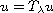. As it can be shown the well known Krasnoselskii's fixed point theorem on cones in the Banach space C[0,1] cannot be applied. More exactly, there is no (positive) value of the parameterfor which the condensing property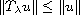, with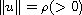is satisfied. To overcome this fact we enlarge the space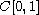and work in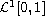where, now, Krasnoselskii's fixed point theorem is applicable. Compactness criteria in this space are, certainly, needed.

Submitted July 20, 2017. Published January 19, 2018.
Math Subject Classifications: 34B10, 34B15.
Key Words: Nonlocal boundary value problem; boundary value problems at resonance; second order differential equations; Krasnoselskii's fixed point theorem on cones.

Show me the PDF file (271 KB), TEX file for this article.George L. Karakostas Department of Mathematics University of Ioannina 451 10 Ioannina, Greece email: gkarako@uoi.gr Konstantina G. Palaska Department of Mathematics University of Ioannina 451 10 Ioannina, Greece email: cpalaska@cc.uoi.gr